Achieve Innovative Process Improvement＋Standardization by IT System with MS 365.Simple Regression Analysis Interpretation (Excel Data Analysis Tools)【Regression Analysis Series 2】\ この記事を共有 /
Simple Regression Analysis Interpret...

This post explains how to interpret results of Simple Regression Analysis using Excel Data Analysis Tools. You’ll learn about the ‘Coefficient of Determination’, ‘Correlation Coefficient’, ‘Adjusted R Square’ and the differences among them.

(Duration: 6:43)

Making a Simple Regression Equation with the Simple Regression Analysis using the Excel Analysis Tool.

Hi, this is Mike Negami, Lean Sigma Black Belt.

We learned about the basics of Regression Analysis and how to get a Single Regression Equation from the Scatter Plot in the previous post. ⇒ “Simple Regression Analysis by Scatter Plot in Excel”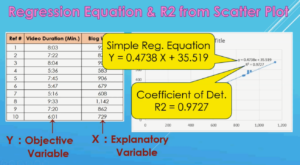Here are the results from the previous Scatter Plot. We obtained a Simple Regression Equation of Y = 0.4738 X + 35.519 and a Coefficient of Determination of 0.9727.

In practice, you can complete your job with only the Scatter Plot, many times, but performing a Single Regression Analysis will give you more significant information. Let’s see the results of a Simple Regression Analysis in Excel with the same data first.There are so many numbers, but you really only need to know the parts in yellow in the image above for business, at least. The numbers in the ‘Coefficients’ column are the exact same numbers as the Coefficient and Intercept in the Regression Equation. (See the image below.) Therefore, you’ll use these numbers to make a Regression Equation.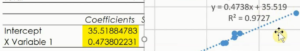P-Value represents the degree of relationship between the Explanatory Variable X and the Objective Variable Y.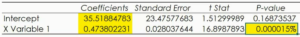Next is the P-Value of the X value. It is 0.000015%. This P-Value is a numerical value which we cannot get from the Scatter Plot. Simply speaking, you can see how well your Explanatory Variable explains the Objective Variable.

In a Multiple Regression Analysis, this P-Value is very important, and you can find which Explanatory Variable among the others has the most influence on the Objective Variable.

For a little more detail, the P-Value is the probability of a null hypothesis. What is that? The null hypothesis in a Regression Analysis is “To assume that the Explanatory Variable of this X is no relation to the Objective Variable of Y”.

Generally, if this percentage is less than 5%, this null hypothesis can be rejected. In other words, “Being no relation” is rejected, which means that there is a lot of relationship between X and Y.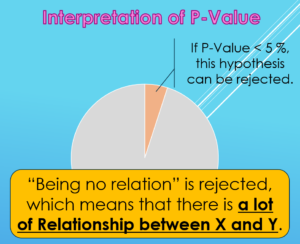In our case, the probability is 0.000015%, which is much less than 5%, so we’ll reject it which means their relationship is significant.

If this P-Value is more than 5%, you need to check the data for errors and add more sample data to redo the analysis or conclude that the two data groups have no relation. In our case it’s less than 5% so I can continue to the next step.

The Differences between ‘Multiple R’, ‘R Square’ and ‘Adjusted R Square’

‘R Square’ and ‘Multiple R’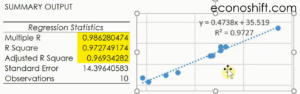Let’s look at the top table. See ‘R Square’. This number is exactly the same as the ‘Coefficient of Determination’ in the Scatter Plot. This is between 0 to 1 and indicates how well the Regression Equation calculates the Objective Variable. Closer to 1 is better.

‘Multiple R’ is the ‘Correlation Coefficient’. You know that squaring it, will be the same as the ‘R Square’.However, I would like you to use the ‘Adjusted R Square’ below as a Coefficient of Determination. It’s a little lower than the ‘R Square’ above. What’s the difference between the two?

In practice, you may need to add more Explanatory Variables and perform the Multiple Regression Analysis. The ‘R Square’ above has the characteristic that the more you have Explanatory Variables, the higher the number becomes, which is mis-leading.

The ‘Adjusted R Square’ below corrects that issue. There is not much influence by it in a Single Regression Analysis, but it would be better to use the ‘Adjusted R Square’ regularly.

I summarized this procedure below:

1. After getting the result of a Single Regression Analysis, confirm that the P-Value of X1 is 5% or less.
2. Look at the value of ‘Adjusted R2’. Depending on your situation, it should be acceptable if it’s 0.5 or more.
3. Then, find a Simple Regression Equation for your data from the X’s Coefficient and Intercept. In our case, it’s Y = 0.4738 X + 35.5188. This makes it possible to predict Y from X of your data.

How to conduct Regression Analysis in Excel

Lastly, I’ll briefly show how to get Single Regression Analysis results from the Excel Data Analysis Tool.  By the way, you would do the same way for a Multiple Regression Analysis too.

Click ‘Data’, ‘Data Analysis Tools’ and select ‘Regression’.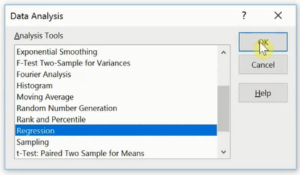In ‘Input Y Range’, you’ll select the data of your Objective Variable, in my case ‘Video Duration’. In the ‘Input X Range’, you’ll select the data of your Explanatory Variable, in my case, it’s ‘Number of Blog Words’ and ‘OK’.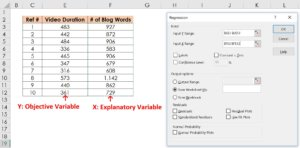If you don’t see ‘Data Analysis Tools’ in the ‘Data’ Ribbon, click ‘File’, ‘Options’ and ‘Add-ins’. After making sure that there is ‘Excel Add-ins’ here, click ‘Go…’.Check ‘Analysis ToolPak’ and click ‘OK’, then it’ll show up.

You can conduct Scatter Plot and Simple Regression Analysis with Excel very easily, so please put some data in and practice them, then you’ll find unexpected discoveries in your business.

“See these other popular articles.”

Comment

1.p61385 says:

It’s amazing designed for me to have a website, which is useful in favor of my